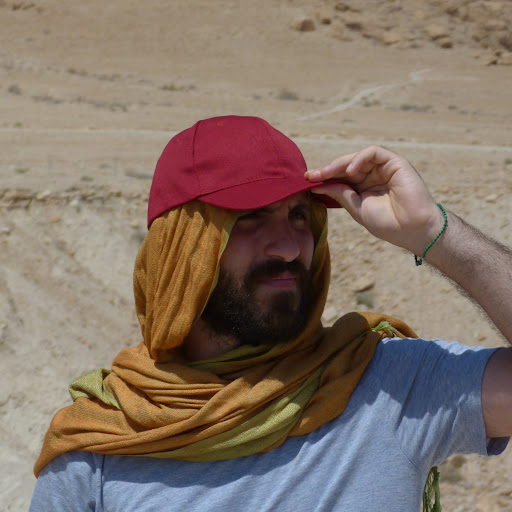1:11 PM
Hi everyone!!! I am looking for an existing name of a construction. Given X simplicial set, how do you call/denote the subcategory of sSet/X with vertices surjections Y-->X ?2:08 PM
You could ask why this should be relevant, so I will explain myself. Under the Grothendieck straightening-unstraightening, simplicial sets X correspond to functors el(X)-->\Delta with (non-empty) set fibers, where the fiber over [n] is morally X_n.

If one wants to study "X-enriched" simplicial sets, that is functors from el(X)^{op} --> Sets, this corresponds to cartesian fibrations C-->el(X), which in turn correspond to simplicial sets Y with a surjection Y-->X. All in all, surjections are associated to functors el(X)^{op} --> Sets.